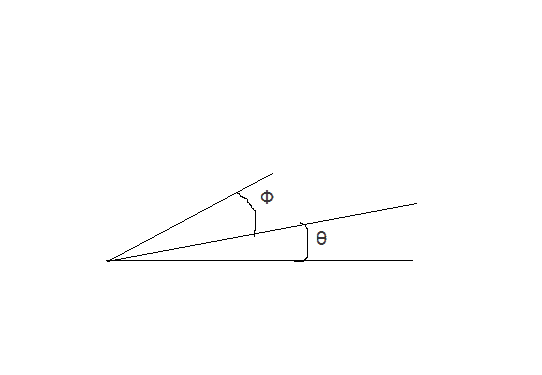# Motion in two dimensions: Launching up an incline

• simphys
I am very very sorry for wasting your time :( my apologies!And so if you look at this: https://www.toppr.com/ask/question/a-projectile-is-fired-up-an-incline-incline-angle-phi-with-an-initial-speed-vi/solving for d yields -> that is the solution but after the last step you did, I cannot translate it in such a way if you know what I mean. (but that is than straight up trigonometry thenn??)Would you want me to post a pic of what I mean...f

#### simphys

Homework Statement
You fire a ball with an initial speed at an angle Φ above the surface of an incline, which is itself inclined at an angle θ above the horizontal (see the above image). (a) Find the distance measured along the incline, from the launch point to the point when the ball strikes the incline. (b) What angle Φ gives the maximum range, measured along the incline? Ignore air resistance.
Relevant Equations
I tried to do this one using

x = y / tan(phi + thetha)
y = v_0sin(phi + theta)t - (gt^2) / 2
x = v_0cos(phi+theta)t => t = x / (v_0cos(phi+theta))But it seems to get too complex and am not sure whether it is correct.. my solution got a bit messy + incorrect

Last edited:
so haven't uploaded it, hope you don't mind.
We don't mind, but then we can't help either. We can't guess what is your problem ...

##\ ##

•simphys
We don't mind, but then we can't help either. We can't guess what is your problem ...

##\ ##

•simphys
Or check out this Insight, Example 3 (Incline landing).

••BvU and simphys
Or check out this Insight, Example 3 (Incline landing).
Toughh i guess I am having problems with the final algebra every x (trig subs actually)

I gave you a link to a thread with a slightly different problem statement (target given, angle to be found).
Your problem statement gives angles and asks for a distance to target along the slope.
My guess is you're off track because you end up with an equation in ##x## that does no longer depend on ##v_0##. That is very strange...

##\ ##

•simphys
•simphys
A real spoiler !
It even tells us where the exercise comes from !
Sadly, only b solved which is the the derivate of a which isn't dolved there, unfortunately:(
I gave you a link to a thread with a slightly different problem statement (target given, angle to be found).
Your problem statement gives angles and asks for a distance to target along the slope.
My guess is you're off track because you end up with an equation in ##x## that does no longer depend on ##v_0##. That is very strange...

##\ ##
or just too complicated because i do have v_0^2 but I forgot to invlude it in the denominator of the quadratic term for y

OKAY! I found this.. The exact same way as i have done!

BUT... the step he immediately calculates d is where I am lost...

it’s amazing how many ways yoj can solve such a problem tough lol

Oh, really ?

BUT... the step he immediately calculates d is where I am lost...
it says (in your notation) $$d\sin\theta = d\cos\theta\tan\alpha - {1\over 2} g \ {d^2\cos^2 \theta\over v_0^2 \cos^2\alpha }$$so of the form ##d(d-z)=0## with non-trivial solution ##d=z##.

##\ ##

•simphys
Oh, really ?

it says (in your notation) $$d\sin\theta = d\cos\theta\tan\alpha - {1\over 2} g \ {d^2\cos^2 \theta\over v_0^2 \cos^2\alpha }$$so of the form ##d(d-z)=0## with non-trivial solution ##d=z##.

##\ ##
yes, the next step was the problem haha, that was the easy step, but then the reformation was not really clear

$$d\sin\theta = d\cos\theta\tan\alpha - {1\over 2} g \ {d^2\cos^2 \theta\over v_0^2 \cos^2\alpha }$$
$$0 = d(\cos\theta\tan\alpha - \sin\theta) - {1\over 2} g \ {d^2\cos^2 \theta\over v_0^2 \cos^2\alpha }$$
So d= 0 or $$(\cos\theta\tan\alpha - \sin\theta) - d \; {g\cos^2 \theta\over 2 v_0^2 \cos^2\alpha }=0$$meaning ##d = ...##

$$d\sin\theta = d\cos\theta\tan\alpha - {1\over 2} g \ {d^2\cos^2 \theta\over v_0^2 \cos^2\alpha }$$
$$0 = d(\cos\theta\tan\alpha - \sin\theta) - {1\over 2} g \ {d^2\cos^2 \theta\over v_0^2 \cos^2\alpha }$$
So d= 0 or $$(\cos\theta\tan\alpha - \sin\theta) - d \; {g\cos^2 \theta\over 2 v_0^2 \cos^2\alpha }=0$$meaning ##d = ...##
Thank you ,I meant this one :) :

## d=g*cos(2ϕ)2*v_i2*cos(θ)[sinθ_icosϕ−sinϕcosθ_i]​

that basically the d =... that you said, I just can't see the damn realtion of it for some reasons from ttrig

yes, the next step was the problem haha,
So next () time don't write 'the next step' but write out the step in question !

Thank you ,I meant this one :) :

## d=g*cos(2ϕ)2*v_i2*cos(θ)[sinθ_icosϕ−sinϕcosθ_i]​

I don't see this appearing anywhere -- wrong dimensions

##\ ##

So next () time don't write 'the next step' but write out the step in question !

I don't see this appearing anywhere -- wrong dimensions

##\ ##
yeah you are totally right, I am very very sorry for wasting your time :( my apologies!
and so if you look at this: https://www.toppr.com/ask/question/a-projectile-is-fired-up-an-incline-incline-angle-phi-with-an-initial-speed-vi/
solving for d yields -> that is the solution but after the last step you did, I cannot translate it in such a way if you know what I mean. (but that is than straight up trigonometry thenn??)

would you want me to post a pic of what I mean ?

or... you know what.. let me not bother you with this exercise, I immensly appreciate the help! Thank you, I think that it is just trigonometry anyways at this point. If I struggle with it ill just do a bit more practice on it and that's it lol